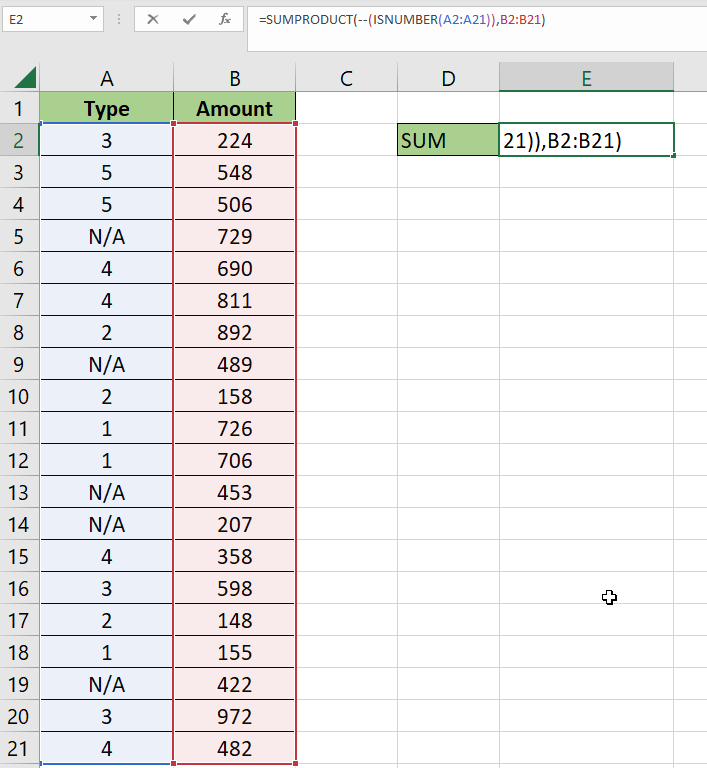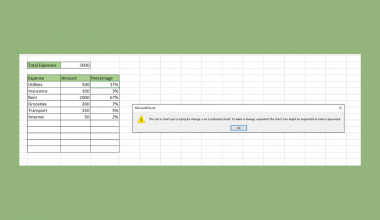# How to Use SUMIF with ISNUMBER in Excel

This guide will explain how to use the SUMIF function only to add numbers in a range if the value in a corresponding field is a number.

We will also explain how to use the SUMPRODUCT and ISNUMBER functions to perform a similar operation.

Finding the sum of a range of values is a common operation performed in an Excel sheet. However, you may want to find the sum of values in a cell range that meets specific criteria. Instead of using the `SUM` function, you must use a function such as `SUMIF` or `SUMIFS`.

Let’s take a look at a quick example of a scenario where you want to find the sum of a range of values where a corresponding range has a numerical value.

Suppose you have a list of transactions made through an online service. Each transaction has a transaction type and a transaction amount. The transaction type can either be a numerical value from 1 to 5 or as the string ‘N/A’.

We want to find the sum of all transaction amounts where the equivalent transaction type is a numerical value.

We can use the `SUMIF` and `SUMPRODUCT` functions to perform this type of conditional summation.

Now that we know what Excel functions we can use to add numbers based on the num, let’s learn how to use them on an actual sample spreadsheet.

## A Real Example of Using SUMIF with ISNUMBER in Excel

The following section provides several examples of how to find the sum of a range using specific criteria. We will also explain the formulas and tools used in these examples.

First, let’s look into our sample data. We have 20 transactions with a corresponding transaction types and amount.We want to return the total amount from transactions with a numerical value as the transaction type.In the example above, we used the following formula to find the total:

`=SUMIF(A2:A21,">="&MIN(A2:A21),B2:B21)`

We can also use the `SUMPRODUCT` to ignore values that have an associated non-numeric value.To get the values in cell E2, we just need to use the following formula:

`=SUMPRODUCT(--(ISNUMBER(A2:A21)),B2:B21)`

Do you want to take a closer look at our examples? You can make your own copy of the spreadsheet above using the link attached below.

If you’re ready to try out the `SUMIF` function with `ISNUMBER`, head over to the next section to read our step-by-step breakdown on how to do it!

## How to Use SUMIF with ISNUMBER in Excel

This section will guide you through each step needed to start using the `SUMIF` function to add all numbers in a range with a corresponding numeric value in another field. You’ll also learn how we can use the `SUMPRODUCT` and `ISNUMBER` functions to achieve the same result.

1. First, we’ll explain how to use the `SUMPRODUCT` and `ISNUMBER` functions together to selectively add a range of numbers.Start by selecting an empty cell to place the formula needed.
2. Type ‘=SUMPRODUCT(‘ to start the `SUMPRODUCT` function. We will use this function to filter out numbers in our sum range based on the value found in a corresponding range.3. We’ll use the formula `--(ISNUMBER(A2:A21)) `to output an array of 1’s and 0’s from a particular range. The ‘–’ symbol converts the boolean values into numbers. This will help us multiply the range with the sum range later on.A ‘1’ indicates that the original cell value is numerical, while a ‘0’ indicates a non-numerical cell value.
4. Next, we will add the range we want to sum as the second argument. Hit the Enter key to evaluate the `SUMPRODUCT` function.5. You should now have the total sum of the specified range where the corresponding value is a number.The `SUMPRODUCT` function multiplies the amounts with the result of the `ISNUMBER` function.
6. Next, we’ll show you another way to sum up a range based on the criteria of a corresponding range being numerical.
First, type ‘=SUMIF(‘ to start the `SUMIF` function. Add the range you want to use as criteria as the first argument of the `SUMIF` function.7. Next, we’ll use the argument “>=”&MIN(A2:A22), as the criteria. This criteria finds the smallest value in the range and compares that value to each value in the first argument.Since non-numerical values will return `FALSE` automatically, this is an effective alternative to using the `ISNUMBER` function within a single formula.
8. For the third argument of `SUMIF`, specify the sum range.Ensure that the sum range is the same length as the first argument.
9. Hit the Enter key to evaluate the function.These are all the steps needed to find the sum of a range based on whether a corresponding range has a numerical value.

This step-by-step guide should provide you with all the information you need to perform a `SUMIF` operation on a range based on whether a corresponding range has a numerical value.

We’ve shown you two possible formulas that you can use to perform this operation. The first formula requires the use of the `SUMPRODUCT` and `ISNUMBER` functions. The `SUMIF` and `MIN` functions are used instead for the second approach.

The `SUMIF` function is just one example of the many Excel functions you can use in your spreadsheets. Our website offers hundreds of other functions and methods to help you get more out of Microsoft Excel.

With so many other Excel functions available, you can find one appropriate for your use case.

Don’t miss out on our team’s new spreadsheet tips, tricks, and best practices. Subscribe to our newsletter to stay updated on the latest guides from us!### Get emails from us about Excel.

Our goal this year is to create lots of rich, bite-sized tutorials for Excel users like you. If you liked this one, you'd love what we are working on! Readers receive ✨ early access ✨ to new content.

##### You May Also Like## How to Select Data for Graphs in Excel

alsoaaaThis guide will explain several ways to select data for graphs in Excel. Excel graphs are an essential…## How to Protect Formulas Without Protecting Worksheet in Excel

This guide will explain how you can protect formulas without protecting worksheets in Excel. You can protect cells…## How to Enable Macros in Excel

This guide will explain how to enable macros in your Excel spreadsheet. Excel macros are a powerful feature…## How to Use SUMIF From Another Sheet in Excel

This guide will explain how to use SUMIF from another sheet in Excel. You may want to use…